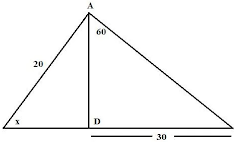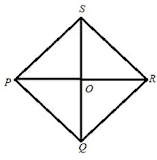# ICSE Solutions for Selina Concise Chapter 24 Solutions of Right Triangles Class 9 Maths

### Exercise 24(A)

1. Fin ‘x’ if:

(i)

(ii)

(iii)

(i) From the figure we have

2. Find angle ‘A’ if:

(i)

(ii)

(iii)

(i) From the figure we have
cos A = 10/20
⇒ cos A = 1/2
⇒ cos A = cos 60°
⇒ A = 60°

(ii) From the figure we have

(iii) From the figure we have
tan A = 10√3/10
⇒ tan A = √3
⇒ tan A = sin 60°
⇒ A = 60°

3. Find angle ‘x’ if:

The figure is drawn as follows :The above figure we have
Again,
⇒ AD = 20 sin x
Now,
20 sin x = 30/√3
⇒ sin x = 30/20√3
⇒ sin x = √3/2
⇒ sin x = sin 60°
⇒ x = 60°

(i)

(ii)

(i) From the right triangle ABE
tan45° = AE/BE
⇒ 1 = AE/BE
⇒ AE = BE
Therefore, AE = BE = 50 m.
Now, from the rectangle BCDE we have
DE = BC = 10 m.
Therefore, the length of AD will be:
AD = AE + DE = 50 + 10 = 60 m.

(ii) From the triangle ABD we have
⇒ sin 30 = AD/100 [Since ∠ACD is the exterior angle of the triangle ABC]

From right triangle ABC,
tan60° = AC/BC
⇒ √3 = AC/40
⇒ AC = 40√3 cm

From right triangle BDC,
tan45° = DC/BC
⇒ 1 = DC/40
⇒ DC = 40 cm

From the figure, it is clear that AD = AC - DC
⇒ AD = 40√3 - 40
⇒ AD = 40(√3 - 1)

6. Find the lengths of diagonals AC and BD. Given AB = 60 cm and BAD = 60o.

We know, diagonals of a rhombus bisect each other at right angles and also bisect the angle of vertex .
The figure is shown below :

Consider the figure:

Given FC = 20, ED = 30,  So EP = 10cm
Therefore,
tan 60° = FP/EP
⇒ √3 = FP/10
⇒ FP = 10√3 = 17.32 cm
Thus, AB = AC + CD + BD = 54.64 cm.

8. In trapezium ABCD, as shown, AB∥DC, AD = DC = BC = 20 cm and A = 60o. Find:

(i) length of AB

(ii) distance between AB and DC.

First draw two perpendiculars to AB from the point D and C respectively. Since AB|| CD therefore PMCD will be a rectangle.
Consider the figure:(i) From right triangle ADP we have
⇒ 1/2 = AP/20
⇒ AP = 10
Similarly from the right triangle BMC we have BM = 10cm .
Now from the rectangle PMCD we have CD = PM = 20 cm .
Therefore,
AB = AP + PM + MB = 10 +20 +10 = 40 cm.
(ii) Again from the right triangle APD we have,
sin 60° = PD/20
⇒ √3/2 = PD/20
⇒ PD = 10√3
Therefore, the distance between AB and CD is 10√3.

From right triangle AQP

10. Find the length of AB.

tan 45° = AE/DE
⇒ 1 = AE/30
⇒ AE = 30 cm
Also, from triangle DBE
tan 60° = BE/DE
⇒ √3 = BE/30
⇒ BE = 30√3  cm
Therefore, AB = AE + BE = 30 + 30√3 = 30(1 + √3) cm

11. In the given figure, AB and EC are parallel to each other. Sides AD and BC are 2 cm each and are perpendicular to AB. Given that AED = 60o and ACD = 45o. Calculate:

(i) AB

(ii) AC

(iii) AE

(i) From the triangle ADC  we have
⇒ 1 = 2/DC
⇒ DC = 2
Since, AD॥DC and AD⊥EC, ABCD is a parallelogram and hence opposite sides are equal.
Therefore, AB = DC = 2cm

(ii) Again,
⇒ 1/√2 = 2/AC
⇒ AC = 2√2

(iii) From the right triangle ADE we have
⇒ √3/2 = 2/AE
⇒ AE = 4/√3

13. Find

(i) BC

(iii) AC

14. In right-angled triangle ABC; B = 90o. Find the magnitude of angle A, if:

(i) AB is √3 times of BC.

(ii) BC is √3 times of AB.

Consider the figure

15. A ladder is placed against a vertical tower. If the ladder makes an angle of 30o with the ground and reaches upto a height of 15 m of the tower; find length of the ladder.

Given that the ladder makes an angle of 30° with the ground and reaches upto a height of 15 m of the tower which is shown in the figure below:

16. A kite is attached to a 100 m long string. Find the greatest height reached by the kite when its string makes an angles of 60o with the level ground.

17. Find AB and BC, if:

(i)

(ii)

(iii)

(i) Let BC = xm
BD = BC + CD = (x + 20)cm
In △ABD,

(ii) Let BC = x m
BD = BC + CD = (x+20)cm
In △ABD,
(iii) Let BC =  x m
BD = BC + CD = (x+20) cm
In △ABD,

18. Find PQ, if AB = 150 m, P = 30o and Q = 45o.

(i)

(ii)

19. If tan xo = 5 /12, tan yo = 3/4 and AB = 48 m; find the length of CD